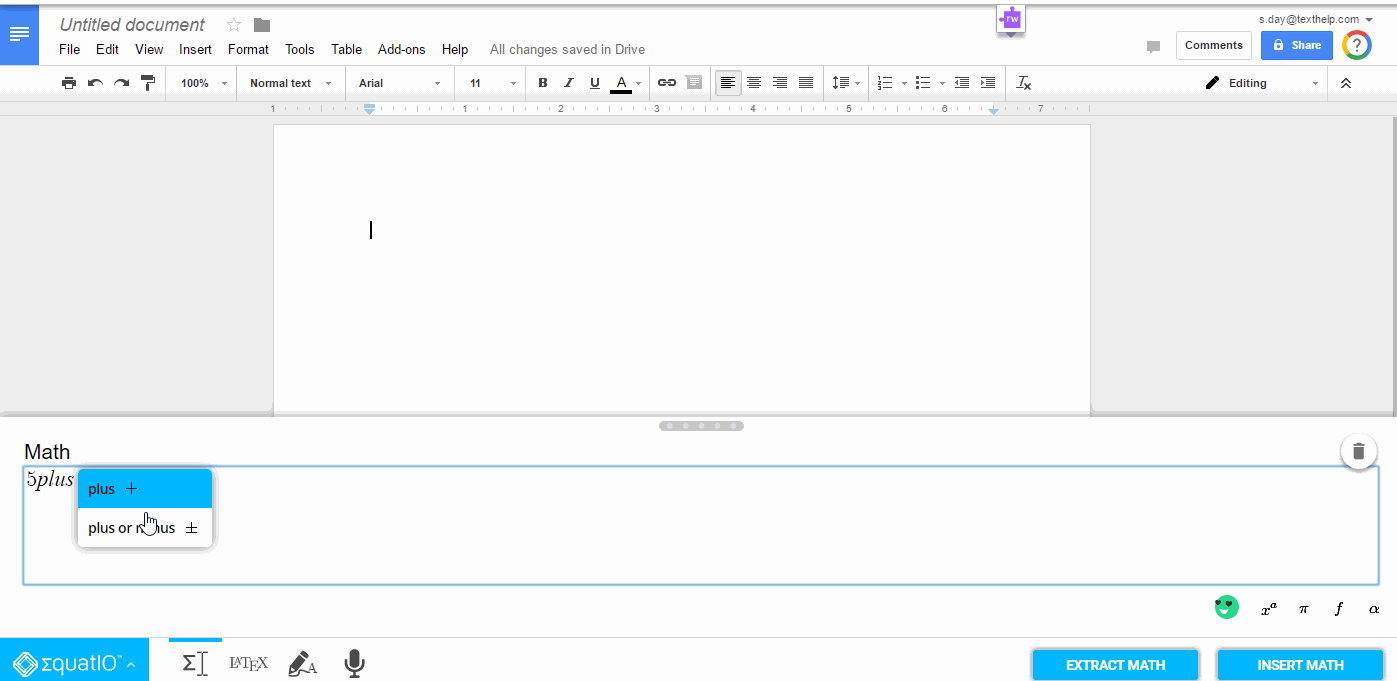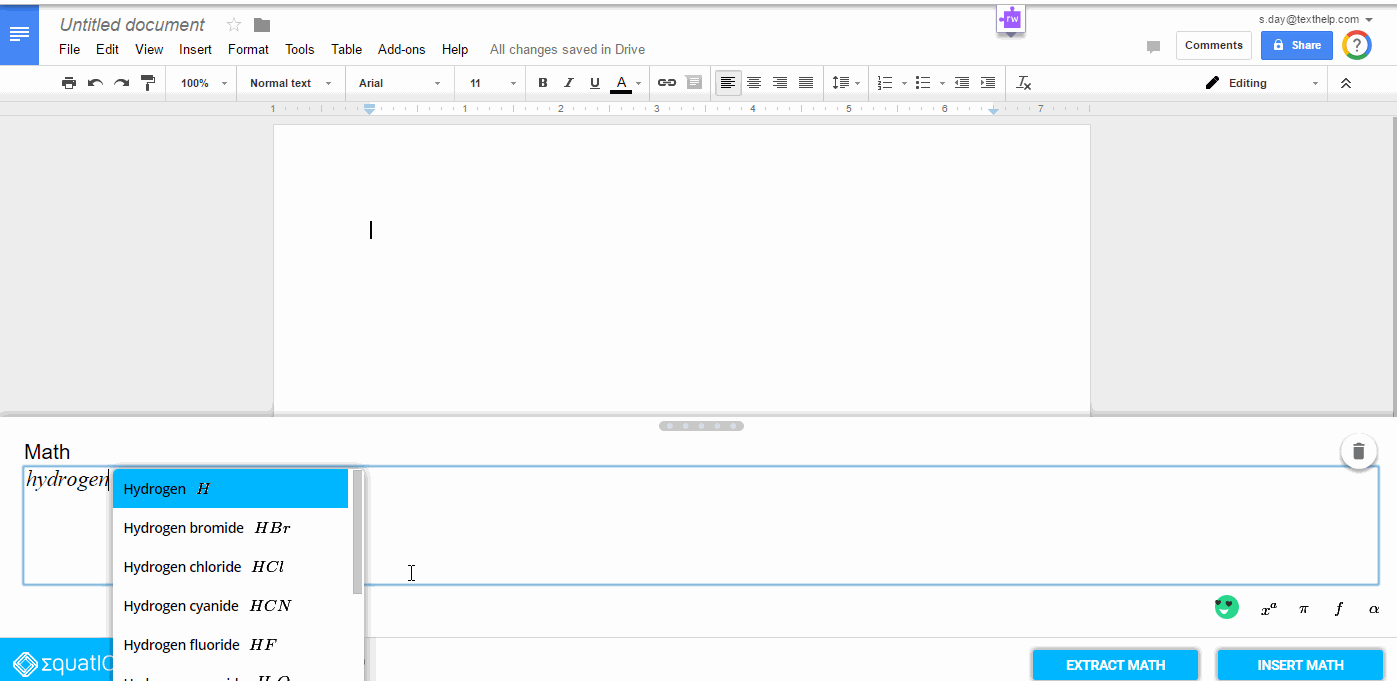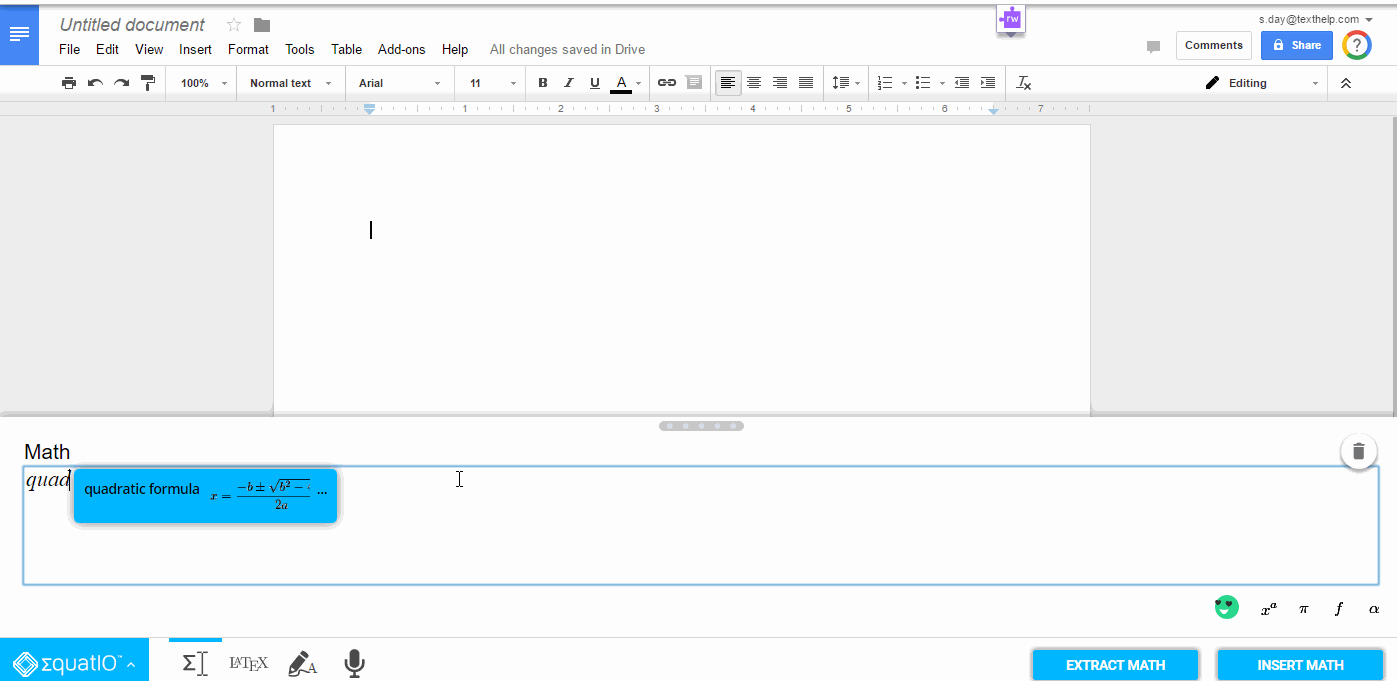# Prediction Options Using Equatio

Equatio has several prediction modes which can be toggled on or off in the Options menu, by selection the Equatio pop-up menu in the bottom left of your screen. Prediction can be found under the Math Options tab.

The first, turned on by default, is Math Prediction, which will predict any mathematical operator you begin typing:The second prediction option is Chemistry Prediction, which will predict any chemical notation you begin typing:The third prediction option is Formula Prediction, which will predict any formula you begin to type, such as the Quadratic Formula:Note: Math Prediction is turned on by default, however you can turn on or off any prediction set you would like to use, whether that be all three at once, or only one. You can customize Equatio’s prediction to fit your needs! If you are doing Chemistry homework for example, you may only want to use Chemistry prediction.